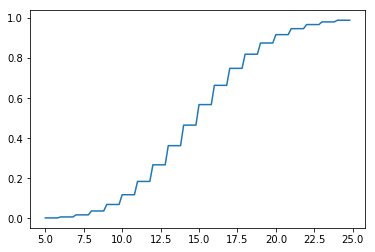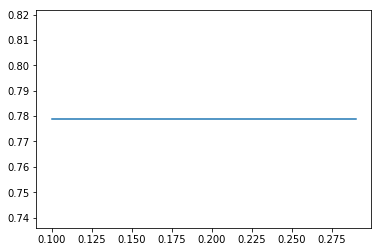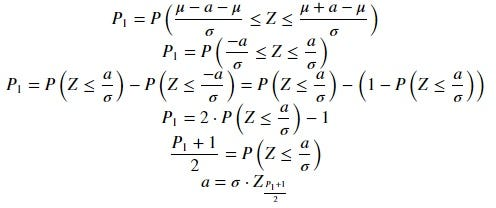Basic Statistics of Events

Appreciating the value of our time

In probability theory, an event is a set of outcomes of an experiment to which a probability is assigned. Those outcomes happen over a period of time, and it is interesting how Python can easily solve the most typical problems.

To better understand how statistical models work, I will illustrate the same case in a way that links the different models. And, considering that the place where I currently work is near a bus stop, I’m going to illustrate the events from something to everyday.

Let’s start from a regular bus line that has to stop at a certain stop bus every 15 minutes.

Perhaps the first idea we could have about a regular bus line is that it is like a periodic impulse response:

Obviously, statistics are based on something more like this:

So, the first question we can make is: what tools we need to guess our results?

Installing libraries

We will work under Python. And it is interesting to install numpy and if you want to draw easy graphics matplotlib. So,

`pip install numpypip install matplotlib`

After doing that, we will work with some namespace:

`import numpy as npimport scipy.stats as st`

Now, let’s consider the first statistic model.

Poisson / Gamma distributions

You just missed the bus, you are at the bus stop, and you have to go to a job interview in 25 minutes. The bus will need 5 minutes, so what is the probability next bus will appear in 20 minutes in a regular line of 15 minutes?

Events happen regularly in μ minutes, what is the probability to the next event in t minutes?

Considering mu=μ and timeMax = t, the next code will use the cumulative distribution of a Poisson to give us the response.

`maxWaitNext = lambda mu: lambda timeMax: st.poisson.cdf(timeMax, mu)`

So, we can use the function in this way:

`>>> timeToWait = 17>>> print("There is %.2f%% next bus will appear in %d minutes "%(round(100*maxWaitNext(15)(timeToWait),2),timeToWait))There is 74.89% next bus will appear in 17 minutes`

The Poisson distribution recognizes all cases that happen with a predictable periodicity within a range (seconds, minutes, days) but without being able to control the quality of the events. In the case of the bus, no matter how good a worker, the driver cannot control the weather, traffic, and so on.

If we want to illustrate how our function works, here I present the code to make a graph

`X = np.arange(5, 25, .2)Y = maxWaitNext(15)(X)fig, ax = plt.subplots()ax.plot(X, Y)plt.show()`Probability to find a bus after waiting X minutes in a regular line of 15 minutes

As you can see, the difference between 15 minutes and 16 minutes could be very important in this graph, but if we study this same result in hours the graph will be a constant:Probability* to find a bus after waiting X hours in a regular line of 15/60 hours

What this means is that scale is important, and each model works under one range. But the best way to change the range is counting groups of events.

The Probability Mass Function of the Poisson model is more appropriate to study the number of events that occur over a period of time. That is, if in an hour and 30 minutes we know 6 buses pass by the stop, what is the probability that exactly 5 buses pass by the stop?

An event happens regularly in time, what is the probability to get exactly K events at the same time?

For the next model we use tipicalEv = A and otherEv = K.

`probEvents = lambda tipicalEv: lambda otherEv: st.poisson.pmf(otherEv, tipicalEv)`

So we can use the code in this way:

`>>> expectedBuses = 5>>> print("There is %.2f%% in a 1 hour 30 min. appear %d buses exactly " %(round(100*probEvents(6)(expectedBuses),2), expectedBuses))`
`There is 16.06% in a 1 hour 30 min. appear 5 buses exactly`

An event happens regularly in μ minutes, how many events will be expected to happen in the t minutes?

In this case, we will use the hope of the model Poisson. Where averageTi = μ and newTi = t.

`expectedInTime = lambda averageTi, tipicalEv: lambda newti: tipicalEv*newTi/averageTi`

If we saw 6 buses passing by in 1 hour and 30 minutes, how many in an hour?

`time = 1print("There will be expected %.0f buses in %d hours"%(round(expectedInTime(1.5, 6)(time),1),time))`

Considering A events happens regularly in μ minutes, what is the probability to the next K events in next t minutes?

• The number of buses which passes by in an hour can be described by a Poisson.
• The time needed to pass by K buses can be described by a Gamma(K, μ/A)

Considering that the next t minutes is cumulative, it is necessary to use its Cumulative Distribution Function with shape is set by a parameter to determine the next events.

`probEvInTime = lambda averageTi, tipicalEv: lambda nextTi, nextEv: st.gamma.cdf(nextTi/(averageTi/tipicalEv), a=nextEv)`

So, if we know it is usual to see 6 buses pass in an hour and a half, what is the probability to see 5 buses in an hour?

`>>> timeToWait = 1>>> print("There is %.2f%% next 5 buses will appear in %d hours "%(round(100*probEvInTime(1.5, 6)(timeToWait, 5),2),timeToWait))There is 37.12% next 5 buses will appear in 1 hours`

Normal distributions

When we are able to accumulate the different events in such a way that we can consider the quality of our work by our management, the techniques we use, etc…, then we can already use a normal distribution.

As soon as it doesn’t make sense to use a normal distribution to find gold ores, it won’t make sense to associate it with catching angler fish. However, fishing with nets could be attributed to a normal distribution, insofar as the average uses massive quantities of fish.

It is for this reason that the management of waiting time at a bus stop may well be studied annually to attribute a quality to it through variance. Less variance better management.

In fact, Python provides easy methods to guess the parameters of our models

`>>> st.norm.fit([15,16,17,14,15,14])(15.166666666666666, 1.0671873729054748)`

Considering the variance is associated with error margin if our management is valued with parameters μ (average of the values), σ (standard deviation), what is the deviation produced under a probability P1?

Value of deviation a for: X ~ N(μ, σ): P1 = P(μ-a ≤ X ≤ μ+a)Requesting for a; X ~ N(μ, σ): P1 = P(μ-a ≤ X ≤ μ+a); Z ~ N(0, 1)

So it is deduced the next code:

`devA = lambda dev, P1 : dev*st.norm.ppf((P1+1)/2)`

Method .ppf is the inverse of the Cumulative Density Function, and the P1 parameter is our tolerance: the proportion of cases we are willing to accept. As you can see, the μ param is not necessary.

Usually, all successful events need μ minutes to happen with a deviation of σ minutes. How many days in a month will happen between timeMin and timeMax?

In this case, we will use the cdf (Cumulative Density Function) to solve the equation days = 30⋅P(minT≤X≤maxT)=P(X≤maxT)−P(X≤minT))

`days = lambda mu, dev, minT, maxT: round(30*(\    st.norm.cdf(maxT, loc = mu, scale = dev) \    - st.norm.cdf(minT, loc = mu, scale = dev)),0)`

At this precise moment, we could procure ourselves a Swiss Army Knife to solve several possibilities. If we have a fleet of N buses which usually pass by mu minutes with a standard deviation of dev, we can construct a function which depends on an interval of time (minT, maxT).

`buses = lambda N, mu, dev: lambda minT, maxT: round(N*(\    st.norm.pdf(minT, loc = mu, scale = dev) if minT==maxT \    else (                                                            st.norm.cdf(maxT, loc = mu, scale = dev) \            if maxT is not None else 1 \        - st.norm.cdf(minT, loc = mu, scale = dev) \            if minT is not None else 0    )    ),1)`

So we can use it for 500 buses whose delay is 15 minutes and 1 of standard deviation:

`f1 = est(500, 15, 1)print("Buses arriving between 10 and 20 minutes: ", f1(10, 20))print("Buses that take more than 30 minutes: ", f1(30, None))print("Buses arriving within 10 minutes: ", f1(None, 10))print("Buses arriving in 15 exact minutes: ", f1(15, 15))`

Weibull / Gumbel / Fréchet distributions

Imagine that we have at our disposal three councilors with experience in transport management. Each councilor has been receiving incidents for their management in their respective cities. Which of the three is the best?

Sometimes incidences are located in the antechamber of a chain failure process (such as the work of an infection in the human body). At other times, such incidents help to dispel the possibility of new incidents (such as registering high scores in speed). And, sometimes, chain failures grow in a controlled way (like heating a machine that works efficiently).

According to the theorem of Fisher-Tippett-Gnedenko, all event produced by an extreme value (like registering volcanic eruptions or pots in the New York Stock Exchange) will converge on one of the three statistical models: Weibull, Gumbel (log-Weibull) or Fréchet (inverse-Weibull).

Conclusion

This first approach may help the programmer to better understand part of the communication between statistical models. However, it should never be forgotten that the statistical study of the data does not ensure the certainty of the results, but rather the convergence of certainty towards safer values.